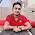## Thursday, 21 February 2013

### C PROGRAM: TO FIND FACTORIAL OF A NUMBER USING POINTER

CODING:
#include<stdio.h>
#include<conio.h>
void main()
{
int num,i,fac,*p1,*p2;
clrscr();
p1=&num;
p2=&fac;
printf("Enter any number ");
scanf("%d",p1);
fac=1;
for(i=1;i<=*p1;i++)
{
*p2=*p2*i;
}
printf("\nFactorial of %d is =  %d",*p1,*p2);
getch();
}CODINGOUTPUT

For any query or suggestion please comment below...

#### 2 comments:

1.Factorial Program in C++

in c++ you can easily write Factorial of any number, it is the product of an integer and all the integers below it for example factorial of 5 is
5! = 5 * 4 * 3 * 2 * 1 = 120. factorial program in c++ is very simple and easy.

2.This article is very clean and useful for using pointers in c Language .
thanks